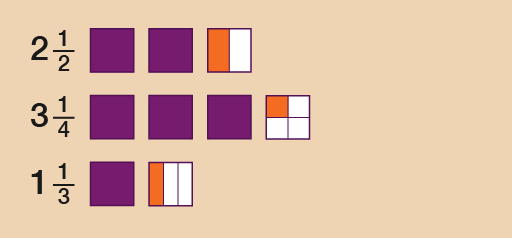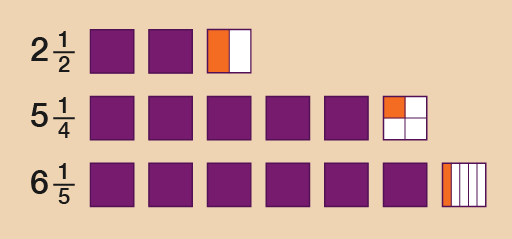Everyday maths 1 (Wales)

Start this free course now. Just create an account and sign in. Enrol and complete the course for a free statement of participation or digital badge if available.

Free course

# 3.2 Drawing fractions

## Example: Drawing the fractions

If you need to compare one fraction with another, it can be useful to draw the fractional parts.

Look at the mixed numbers below. (A mixed number combines a whole number and a fraction.) Say you wanted to put these amounts in order of size, with the smallest first:

2 , 3 , 1

### Method

To answer this you could look at the whole numbers first and then the fractional parts. If you were to draw these, they could look like this:Figure 16 Drawing the fractions

So the correct order would be:

1 , 2 , 3

## Activity 20: Putting fractions in order

1. Put these fractions in order of size, smallest first:
• 5 , 6 , 2
1. Put these fractions in order of size, smallest first:
• 2 , 1 , 2

1. The correct order would be:
• 2 , 5 , 6
• In this case, even though is bigger than and is bigger than , you need to look at the whole numbers first and then the fractions. The diagram illustrates this more clearly:Figure 17 Drawing the fractions
1. The correct order would be:
• 1 , 2 , 2
• Looking at the whole numbers, 1 would be the smallest because the other two mixed numbers are both greater than 2. To see which is bigger out of 2 or 2 , you need to compare the fraction part. Which is bigger: or ?
• To work this out, you could draw images as above, or you could use the method we looked at earlier where you change to equivalent fractions – the bottom numbers of the fractions are 5 and 2, and the lowest common multiple of 5 and 2 is 10:
• 5 × 2 = 10
• 2 × 5 = 10
• Whatever you do to the bottom, do to the top:
• If you mulitiply the top and bottom numbers in by 2, you make .
• If you mulitiply the top and bottom numbers in by 5, you make .
• () is smaller than (), so 2 is smaller than 2.
FSM_1_CYMRU#### Live in Wales? Free your ambition with a paid part-time course with the OU in Wales.

With grants of up to £4,500* to help with living costs, and tuition fee loans to cover course fees,
now's the time to take that next step.

#### Find out more

*Eligibility rules apply for financial support.